Latest SSC jobs   »   Circle: Definition, Circumference, Area, And Examples;...

What is a Circle? A Circle is a 2-dimensional round shape consisting of points at equidistant from the centre. Simply, a circle is a closed curve that divides a plane into 2 regions- interior and exterior. An exact round shape is the perfect example of a circle. Questions based on circles are widely asked in various competitive examinations where candidates need to apply the formula and derive the answer. We are providing you with the important terminologies, formula and examples based on Circles. Download the PDF for notes on Circle with examples.

### Circle Terminology

Arc: Every connected point on the circle is the arc. Determining two endpoints on the arc and centre allows two arcs to form a complete circle together.

Centre: The point that is equidistant from all points on the circle.

Chord: A linear segment dividing the circle into two segments whose endpoints lie on the circle.

Diameter: The greatest distance between two points in a circle is the diameter. It is the longest chord for a circle and is twice as long as the radius.

Radius: A line segment joining the centre of a circle to any point on the circle. It is half of the diameter.

Sector: A region bounded by two radii of equal length with a common centre.

Segment: The region bounded by a chord and one of the arcs connecting the chord’s endpoints.

Semicircle: It is the arc determined by the endpoints of a diameter, taking its midpoint as the centre. The half-disc of the circle is Semicircle.

Tangent: A coplanar straight line intersecting the exterior of the circle at a single point.

Click here for SSC CGL Tier 2 Free Quizzes of all Topics

### Circumference of Circle

The circumference is the distance covered to complete one circle also known as the perimeter of circle. The circumference of a circle is derived by the formula:

### C=2πr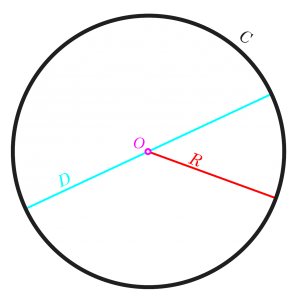### Area of Circle

The area of a circle is determined with the help of the radius of the circle. To derive the area of a circle formula is given by:

## Circle / वृत्त

### Properties of a Circle

The properties of a circle will include the following:

• Each point on the circumference of a circle is at equidistant from the centre.
• The diameter of the circle divides it into 2 equal parts
• Circles that have equal radii are congruent to each other.
• Circles that are different in size or having different radii are similar.
• The diameter of the circle is the largest chord and is twice the radius.

##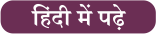#### Congratulations!General Awareness & Science Capsule PDFIncorrect details? Fill the form again here

General Awareness & Science Capsule PDF

Thank You, Your details have been submitted we will get back to you.
•Upcoming Government Jobs 2022, List for ...
•Calendar for Upcoming Government Exams 2...
•RRB NTPC CBT 2 Admit Card 2022, City Wis...
•RRB NTPC Result 2021 Out, CBT 1 Revised ...
•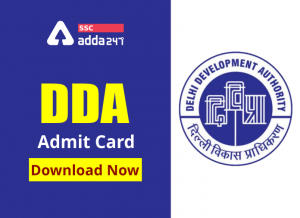DDA Admit Card 2022 Out, Direct Link for...
•SSC CGL Application Status Out for Dest ...
•World Organ Donation Day 2022, History a...
•SSC CPO Admit Card 2022
•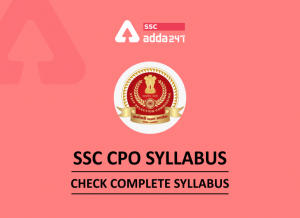SSC CPO Syllabus 2022 and Exam Pattern f...
•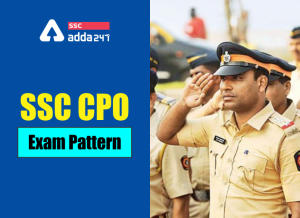SSC CPO Exam Pattern 2022, Check Exam Pa...